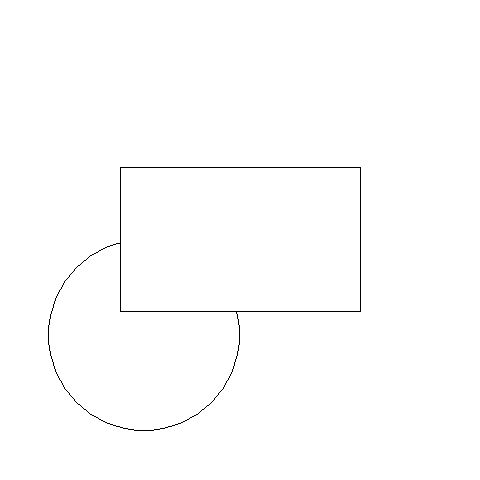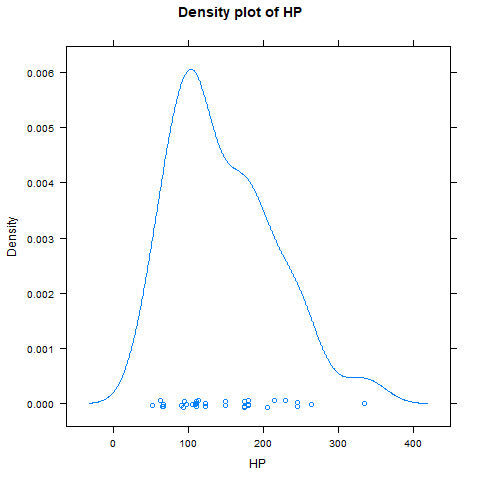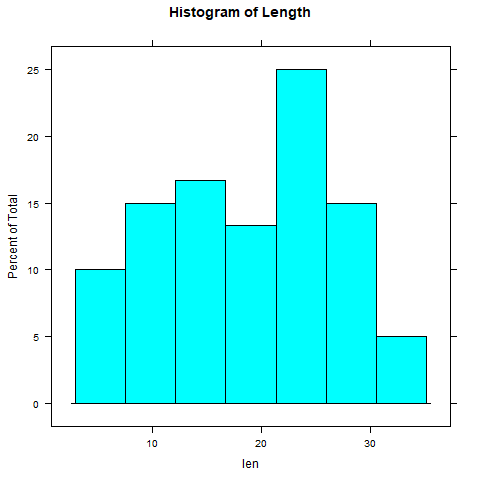GFG App
Open AppBrowser
Continue

# Grid and Lattice Packages in R Programming

Every programming language has packages to implement different functions. Many functions are bundled together in a package. To use those functions, installation and loading of these packages are required. In R programming, there are 10, 000 packages in the CRAN repository. Grid and Lattice are some of the packages in R Programming Language which implement graphical functions to develop some graphical outputs such as rectangle, circle, histogram, barplot, etc.

## R – Grid Package

Grid package in R Programming Language has been removed from CRAN repository as it is now available as the base package. This package is a basis for all other higher graphical functions used in other packages such as lattice, ggplot2, etc. Also, it can manipulate the lattice outputs. Being a base package, there is no requirement to install it. It is automatically installed when R is installed.

To load the grid package, use the below command in console and select “grid” when menu appears:

local({pkg <- select.list(sort(.packages(all.available = TRUE)), graphics=TRUE) + if(nchar(pkg)) library(pkg, character.only=TRUE)})

grid package uses functions circleGrob, linesGrob, polygonGrob, rasterGrob, rectGrob, segmentsGrob, legendGrob, xaxisGrob, and yaxisGrob to create graphical objects (grobs).

To see the list of all the functions in grid package, use the below command:

`library(help = "grid")`

Below is an implementation of some functions on-grid package.

Example:

## R

 `library``(grid)`   `# Saving output as png file` `png``(file =``"grid-gfg.png"``)`   `# Create circle grob` `cir <- ``circleGrob``(name = ``"cir"``, x = 0.3, y = 0.3, r = 0.2,` `gp = ``gpar``(col = ``"black"``, lty = 3))`   `# Draw grob` `grid.draw``(cir)`   `# Create rectangular grob` `rect <- ``rectGrob``(name = ``"rect"``, x = 0.5, y = 0.5,` `width = 0.5, height = 0.3)`   `# Draw grob` `grid.draw``(rect)`   `# Saving the file` `dev.off``()`

Output:## R – lattice Packages

The lattice package uses grid package to provide better relationships between the data. It is an add-on package for the implementation of Trellis graphics (graphics that shows relationships between variables conditioned together).

### To install Lattice Packages in R

`install.packages("lattice")`

There are so many graphs present in the package such as barchart, counterplot, densityplot, histogram, etc. A simple format to use is:

`graph_type(formula, data)`

where,

• graph_type represents the type of graph to represent
• formula specifies the variables or conditioned variables

To know about all functions of package:

`library(help = "lattice")`

Below is an implementation of some of the graphical functions in lattice package.

Example 1:

## R

 `library``(lattice)`   `attach``(mtcars)`   `# Output to be present as PNG file` `png``(``"DensityPlotLatticeGFG.png"``)`   `# Create Density Plot for HP` `densityplot``(~hp, main =``"Density plot of HP"``, xlab =``"HP"``)`   `# Saving the file` `dev.off``()`

Output:Example 2:

## R

 `library``(ToothGrowth)`   `# Output to be present as PNG file` `png``(``"HistogramLatticeGFG.png"``)`   `# Using ToothGrowth dataset` `# To Create Histogram of length` `histogram``(~len, data = ToothGrowth,` `main = ``"Histogram of Length"``)`   `# Saving the file` `dev.off``()`

Output:My Personal Notes arrow_drop_up